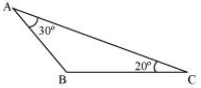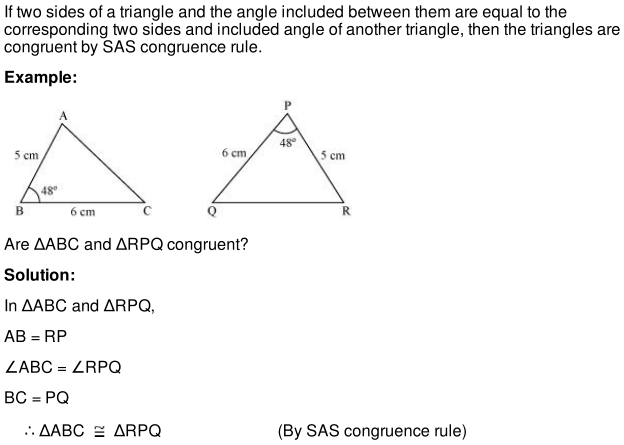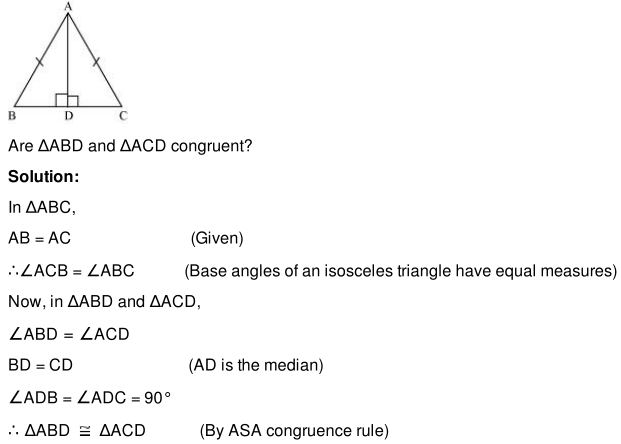## Revision Notes of Ch 7 Triangles Class 9th Math

Topics in the Chapter

• Properties of triangle
• SAS congruence rule
• Meaning of CPCT
• ASA congruence rule
• AAS congruence rule
• SSS congruence rule
• RHS congruence rule

Properties of triangle

• If two sides of a triangle are unequal then the longer side has the greater angle opposite it. Thus, we can say that angle opposite to the shorter side of a triangle is smaller.

For example, in the given triangle, AC > AB, therefore ∠ABC > ∠ACB.• If two angles of a triangle are unequal then the greater angle has the longer side opposite it.Thus, we can say that the smaller angle has the shorter side opposite it.
For example, in the given figure, ∠BAC > ∠ACB, therefore BC > AB.Angles opposite to equal sides of a triangle are equal.

Find the missing angles in the following triangles.Solution

We know that angles opposite to equal sides of a triangle are equal.
∴∠ABC = ∠ACB = x (say)
By angle sum property of triangles, we obtain
∠ABC + ∠BCA + ∠CAB = 180°
⇒ x + x + 70° = 180°
⇒ 2x = 110°
⇒ x = 55°
Thus, ∠ABC = ∠BCA = 55°

SAS congruence ruleMeaning of CPCT

CPCT stands for ‘corresponding parts of congruent triangles’. ‘Corresponding parts’ means corresponding sides and angles of triangles. According to CPCT, if two or more triangles are congruent to one another, then all of their corresponding parts are equal.

ASA congruence rule

If two angles and included side of a triangle are equal to the two corresponding angles and the included side of another triangle, then the triangles are congruent by ASA congruence rule.

Example:
In the following figure, AD is the median of ΔABC.AAS congruence ruleSSS congruence rule

If three sides of a triangle are equal to the three sides of the other triangle, then the two triangles are congruent by SSS congruence rule.

Example:RHS congruence rule

If the hypotenuse and one side of a right triangle are equal to the hypotenuse and one side of the other right triangle, then the two triangles are congruent to each other by RHS congruence rule.